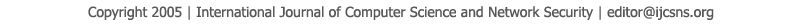To search, Click below search items.All Published Papers Search Service
 Title Author Year Keyword
 Title Complexity Reduction of the Baby-Step Giant-Step Algorithm Author Fahad Alhasoun, M. A. Matin Citation Vol. 11  No. 5  pp. 210-216 Abstract The security of cryptosystems depends on the hardness and difficulty of solving the discrete log problem. One of the well known algorithms to solve the discrete log problem is the Baby-Step Giant-Step Algorithm. This algorithm has √n space and time complexities in the cyclic multiplicative group Zn. In this paper, a modified algorithm is proposed to reduce the space complexity by 50% the algorithm uses properties of 0 function in order to decide what elements are useless for the algorithm. The proposed algorithm of the Baby-Step Giant-Step Algorithm has n time complexity and n/2 space complexity to find a solution for the discrete log problem in the cyclic multiplicative group Zn. The proposed algorithm can find a solution with time complexity of n/2 if it runs with a space complexity of n meaning it will run twice as fast as the conventional algorithm with the same space complexity. Keywords Cryptosystems, cryptography, discrete log problem, Baby-Step Giant-Step Algorithm, Cyclic Multiplicative Groups URL http://paper.ijcsns.org/07_book/201105/20110531.pdf